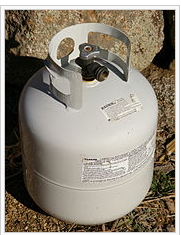## Expressing the Equilibrium Constant of a Gas in Terms of Pressure

#### Learning Objective

• Write the equilibrium expression, KP, in terms of the partial pressures of a gas-phase reaction

#### Key Points

• According to the ideal gas equation, pressure is directly proportional to concentration, assuming volume and temperature are constant.
• Since pressure is directly proportional to concentration, we can write our equilibrium expression for a gas-phase reaction in terms of the partial pressures of each gas. This special equilibrium constant is known as KP.
• KP takes the exact same form as KC. To avoid confusion between the two, do not use brackets ([ ]) when expressing partial pressures.

#### Terms

• equilibriumThe state of a reaction in which the rates of the forward and reverse reactions are the same.
• partial pressureThe pressure that one component of a mixture of gases contributes to the total pressure.

## Equilibrium Constants for Gases

Up to this point, we have been discussing equilibrium constants in terms of concentration. For gas-specific reactions, however, we can also express the equilibrium constant in terms of the partial pressures of the gases involved. Take the general gas-phase reaction:

$aA(g)+bB(g)\rightleftharpoons cC(g)+dD(g)$

Our equilibrium constant in terms of partial pressures, designated KP, is given as:

$K_P=\frac{P^c_CP^d_D}{P^a_AP^b_B}$

Note that this expression is extremely similar to KC, the equilibrium expression written in terms of concentrations. In order to prevent confusion, do not use brackets ([ ]), when writing KP expressions.

## KP and the Ideal Gas Law

The reason we are allowed to write a K expression in terms of partial pressures for gases can be found by looking at the ideal gas law. Recall that the ideal gas law is given by:

$PV=nRT$

Re-writing this expression in terms of P, we have:

$P=\frac{n}{V}RT$

Note that in order for K to be constant, temperature must be constant as well. Therefore, the term RT is a constant in the above expression. As for n/V (moles per unit volume) this is simply a measure of concentration. Pressure is directly proportional to concentration, so we are justified in our use of KP.

Lastly, there is a very important equation that relates KP and KC. It is given as follows:

$K_{p} = K_{c}(RT) ^{ \Delta n}$

In this expression, $\Delta n$ is a measure of the change in number of moles of gas in the reaction. For instance, if a reaction produces three moles of gas, and consumes two moles of gas, then $\Delta n=(3-2)=1$.Liquefied gasInside this tank, propane is compressed into a liquid, which is in equilibrium with its gaseous headspace. The internal pressure of the gaseous propane is a function of temperature.